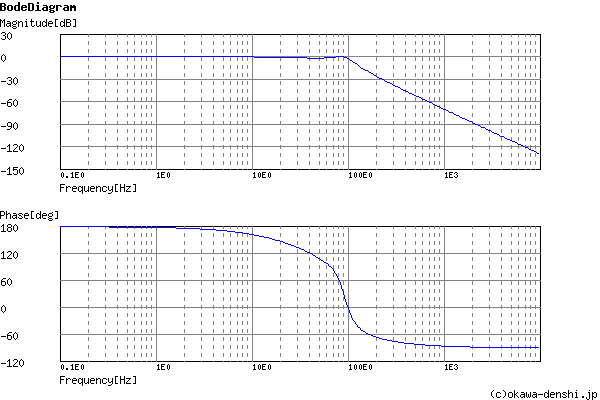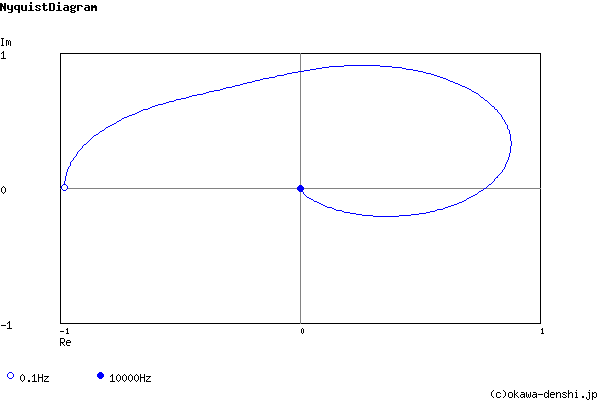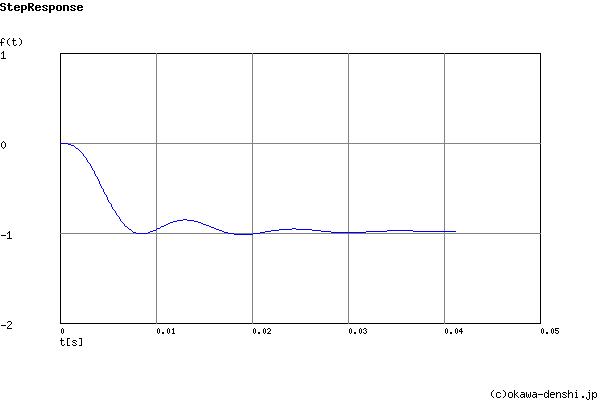# (Sample) 3rd order Multiple Feedback Low-pass Filter Design Tool - Result -

Calculated the Transfer Function for the 3rd order Multiple Feedback Low-pass filter, displayed on graphs, showing Bode diagram, Nyquist diagram, Impulse response and Step response.

## 3rd order Multiple Feedback Filter

 Vi→→Vo
(Sample)Transfer Function:
 G(s)= -79063161.7478 s3+466.724312356s2+392148.494742s+80593959.1348

R1 = 910Ω
R2 = 47kΩ
R3 = 130kΩ
R4 = 47kΩ
C1 = 4.7uF
C2 = 0.22uF
C3 = 0.0022uF

#### Equivalent block diagram:

 Vi(s)→ 2πfc1 s+2πfc1 → (2πfc2)2 s2+2ζ(2πfc2)s+(2πfc2)2 →Vo(s)
Cut-off frequency fc1, fc2 of equivalent block diagram:
fc1 = 37.9811225476[Hz]
fc2 = 92.4905889032[Hz]
Damping ratio ζ of equivalent block diagram:
ζ = 0.196238121389

#### Pole(s)

p = -18.1501794125 +90.6922269158i[Hz]
|p|= 92.4905889032[Hz]
p = -37.9811225476[Hz]
|p|= 37.9811225476[Hz]
p = -18.1501794125-90.6922269158i[Hz]
|p|= 92.4905889032[Hz]#### Phase margin

pm= 214[deg] (f =89[Hz])

#### Oscillation frequency

f = 90.6922269158[Hz]

#### Overshoot (in absolute value)

The 1st peak  gpk = -1.01 (t =0.0085[sec])
The 2nd peak  gpk = -0.85 (t =0.013[sec])
The 3rd peak  gpk = -1.02 (t =0.019[sec])

#### Final value of the step response (on the condition that the system converged when t goes to infinity)

g(∞) = -0.981006053016

Filter gain at f=0Hz:
K=Times (K<0)

### Select filter type

Set parameters of the equivalent block diagram
 1st filter: fc1=Hz 2nd filter: fc2=Hz Damping ratio ζ=

Butterworth filter
Cut-off frequency fc=Hz

Chebyshev filter
Characteristic frequency: fc=Hz
Gain ripple: gr=dB

 C1 = F C2 = F
C1, C2 is optional. But when setting these capacitances, C1 and C2 of both are needed.

Select Capacitor Sequence:
Select Resistor Sequence:

### Frequency analysis

Bode diagram
Phase  Group delay
Nyquist diagram
Pole, zero
Phase margin
Oscillation analysis
Analysis on frequency range:
f1=∼f2=[Hz] (optional)

### Transient analysis

Step response
Impulse response
Overshoot
Final value of the step response
Analysis on time range:
0∼[sec] (optional)

# Frequency analysis# Transient analysis